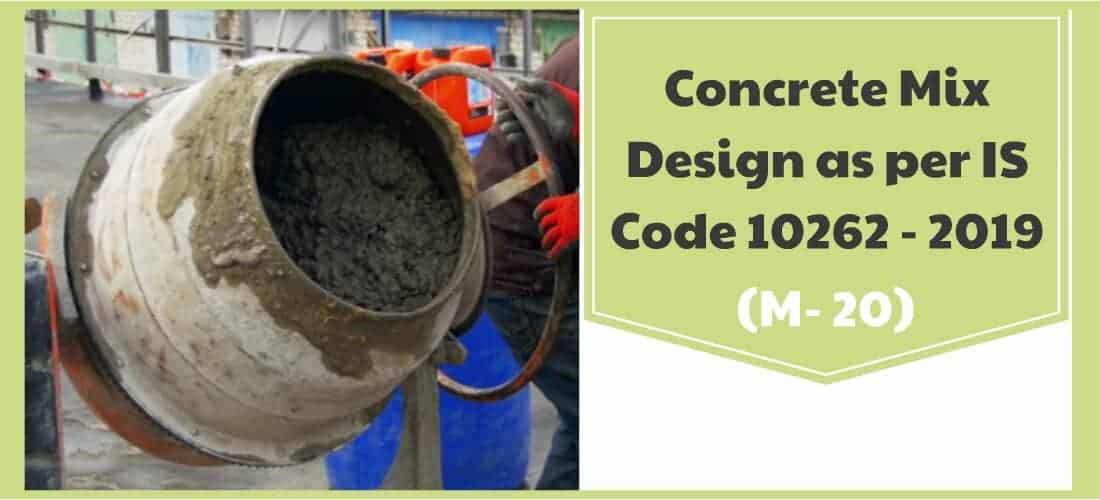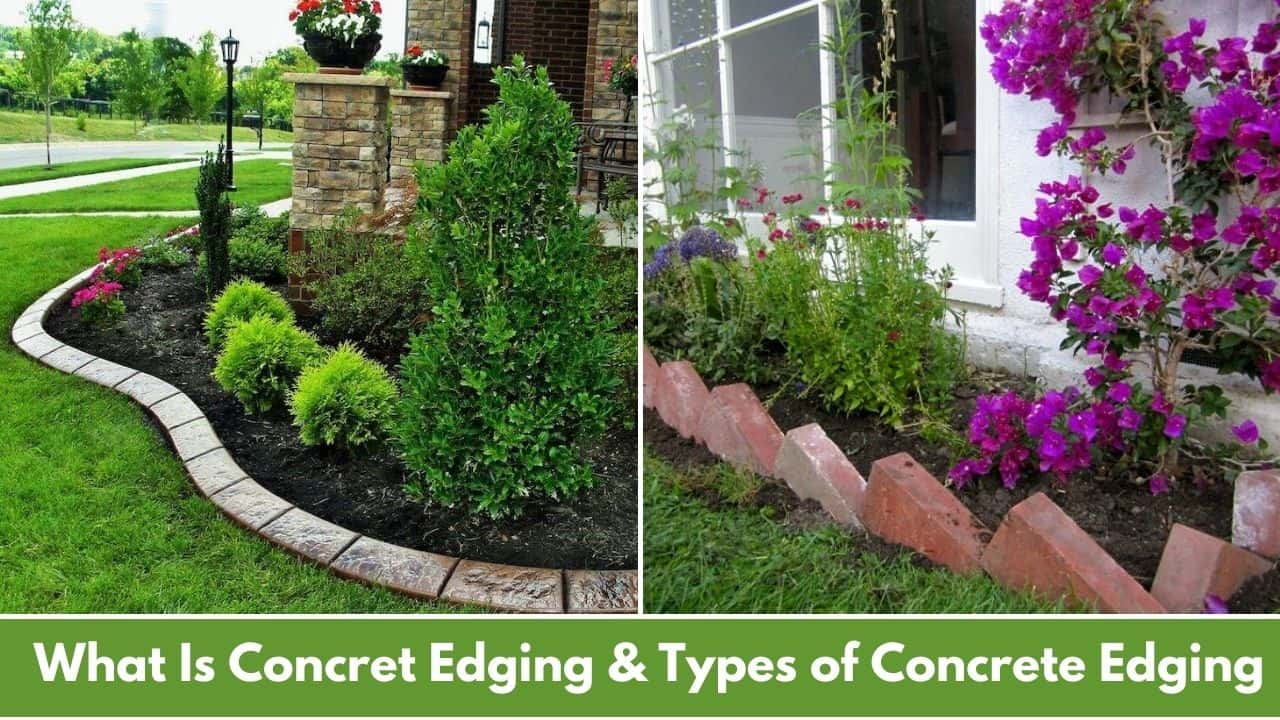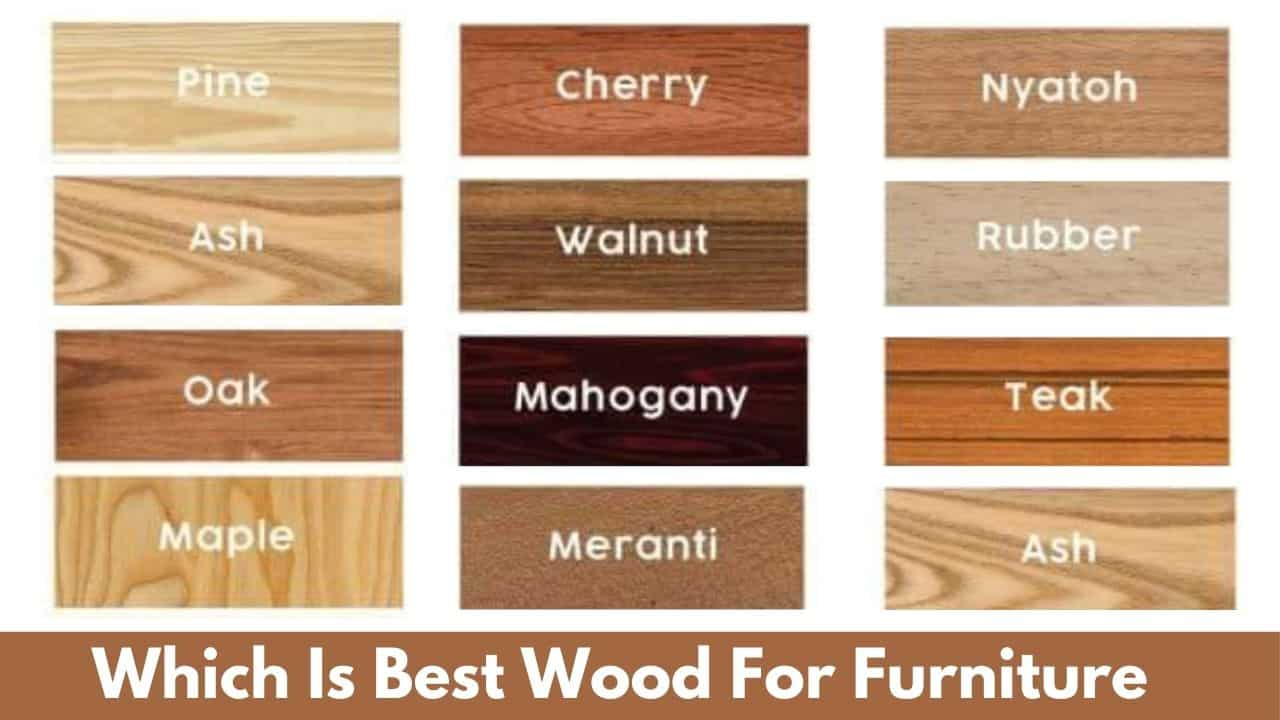# Concrete Mix Design | Concrete Mix Design Calculations pdf | Concrete Mix Design Excel Sheet | IS 10262 Code 2019 pdf Download | Mix Design Code15 min read

Post Contents

## What Is Concrete Mix Design?

Concrete Mix Design can be defined as the method of calculating a suitable quantity of materials of concrete and find out the required proportions with the object of producing concrete of certain minimum strength and durability as economically as possible.

Mix Design of Concrete is a process of calculating the quantity of materials like cement, sand, aggregate, water, and admixtures to achieve or make specified strength of concrete.

In simple words, the method of calculating materials quantity that makes the required grade of concrete.

The concrete mix design is no easy task considering the widely varying properties of the constituent materials, the conditions that prevail at the site of work, in particular the exposure condition, and the conditions that are essential for different construction work for which the mix is designed.

Design of concrete mix demands deep knowledge of concrete materials properties; these make the task of mix design more complex and difficult.

The design process of concrete mix required deep knowledge of material properties and properties of concrete in plastic condition; it also needs wider knowledge and experience of concrete.

Even the quantity of materials of concrete calculated in the laboratory requires modification and readjustments to suit the field conditions.

The advanced research and experiment with deep studies of properties of materials, concrete is becoming more and more an exact material than in the past.

The structural designer considers some minimum criteria for strength for design; and the concrete designer with the knowledge of the materials, site exposure conditions and standard of supervision on construction site to achieve this minimum strength and durability.

Further, it’s the site engineer’s responsibility to make concrete at the site and for that, he has to closely following the parameters suggested by the mix designer to achieve the minimum strength specified by the structural engineer.

In some cases, concrete mix design calculation parameters have to slightly modify the mix proportions given by the mix designer.

There must be the provision of casting concrete cubes and cylinders in required numbers and tests them to confirm the achievements with respect to the minimum specified strength.

Concrete Mix Design Calculation as per IS Code means the Government of Indian releases guidelines for concrete strength and quality control.

Indian standard code gives proper guidelines and methods for designing concrete mix to get proper strength of concrete.

## Concrete Mix Design

The Bureau of Indian Standards recommended a set procedure for the design of concrete mix mainly based on the work done in national laboratories.

The Concrete Mix Design procedure is covered in IS 10262 2019. The methods can utilize for both medium strength and high strength concrete.

The guidelines for concrete mix design is 10262 is 2019 pdf

Data Required for Mix Proportioning

The following data is required for Concrete Mix Design Calculations of a particular grade of concrete.

2. Type of cement, and grade of cement (if applicable)
3. Maximum nominal size of aggregate.
4. Minimum cement/cementitious materials content and maximum watercement/cementitious materials ratio to be adopted; (Exposure conditions as per Table 3 and Table 5 of IS 456)
5. Workability required at the time of placement
6. Transportation time
7. Method of Placing
8. Degree of site control (good/fair) or value of established standard deviation, if any;
9. Type of coarse aggregate (angular/sub-angular/ gravel with some crushed particles/rounded gravel/manufactured coarse aggregate)
10. Type of fine aggregate (natural sand/ crushed stone or gravel sand/manufactured sand/ mixed sand);
11. Maximum cement content
12. Whether a chemical admixture shall or shall not be used and the type of chemical admixture and the extent of use;
13. Whether a mineral admixture shall or shall not be used and the type of mineral admixture and the extent of use; and
14. Any other specific requirement like early age strength requirements.

Following are the steps to be followed for concrete mix design,

Concrete Mix Design for M – 20 Grade of Concrete

Following steps for concrete mix design procedure as per IS Code 10262 – 2019,

### Step-1 Calculate Target Mean Strength of Concrete

In order that not more than the specified proportion of test results are likely to fall below the characteristic strength, the concrete mix has to be proportioned for higher target mean compressive strength f ’ck.

The Target mean characteristic strength is given by the following relation:

f’ck = fck + 1.65 S

or

f’ck = fck + X

whichever is higher.

where,

f’ck = Target mean strength (compressive) at end of 28 days, in N/mm2;

fck = characteristic strength (compressive) at end of 28 days, in N/mm2;

S = standard deviation, in N/mm2 (see 4.2.1); and

X = Factor as per Grade of Concrete, as per Table 1.

Table – 2 Assumed Standard Deviation

For M -20 Grade of Concrete,

Target Mean Compressive Strength (f’ck) = fck + 1.65 S

= 20 + 1.65 x 4 (S = 4.0 for M – 20)

= 26.6 N/mm²

Target Mean Strength ( f’ck) =  fck+ X

= 20 + 5.5

= 25.5 N/mm²

Always select a higher value. Therefore, for the M-20 grade of concrete, we will try to achieve 26.6 N/mm² strength.

### Step-2 Selection of Water-Cement Ratio

It is stated for concrete mix design as per is code that in general different types of cement, aggregates of various maximum size and supplementary cementitious materials, grading, surface texture, shape, and other characteristics may produce concrete of different compressive strength for the same free watercement ratio.

Therefore, a proper relationship between the water-cement ratio and the strength of concrete should preferably be established for the materials actually to be used.

In case such data is not available, related water-cement ratio (by mass) (w/c) corresponding to the compressive strength at 28 days may be selected from the relationship shown in Fig.1, for the expected 28 days strength of cement.

So, for the M – 20 Grade of concrete, we have a target mean strength of 26.66 N/mm² and we have OPC – 53 grade of cement.

As per our cement grade OPC -53, we have to follow Curve 3.

From the graph, for 26.66 N/mm² concrete strength at 28 days bisect the curve 3 at 0.62 free water-cement ratios.

Where supplementary cementitious materials are used, that is, mineral admixtures, the water cementitious materials ratio (w/cm) shall be calculated, in accordance with Table 5 of IS 456 and this w/cm shall be as per Table 3 and Table 5 of IS 456 or as specified.

So, we select water-cement ratio for M- 20 grade of concrete is 0.62.

Suggestion:

Always prefer a higher watercement ratio for your concrete because IS code tests and studies are done in a controlled manner in laboratories which procedure and care are not possible on construction sites. So, my suggestion is to select a higher water-cement ratio for your concrete grade. (Higher means, for M -20 grade of concrete IS code indicates 0.62, select 0.5 less that is 0.55)

### Step-3 Estimation of Air Content

The approximate volume of entrapped air content to be expected in normal (non-air-entrained) concrete is given in Table 3.

We have a nominal maximum size of aggregate of 20 mm.

From the above table, the approximate air content as a percentage of the volume of concrete is 1 %.

### Step-4 Selections of Water Content and Admixture Content

The water content in concrete is generally affected by various factors, such as aggregate size, aggregate shape, aggregate texture, workability, water-cement ratio, cement, and other supplementary cementitious materials type and content, chemical admixture, and environmental conditions.

As aggregate maximum size increases, a reduction in watercement ratio and slump, and the use of rounded aggregate and water reducing admixture will reduce the water demand.

On another side, if the cement content, slump, watercement ratio, aggregate angularity, temperature, and a decrease in the proportion of the coarse aggregate to fine aggregate will increase water demand.

The amount of water content per unit volume of concrete may be determined from Table 4.

The water content given in Table 4 is for angular coarse aggregate and for 50 mm slump.

The water content given in Table 4 can be reduced by approximately 10 kg for subangular aggregates, 15 kg for gravel with some crushed particles, and 20 kg for rounded gravel to produce the same workability.

So, if required more workability means slump value more than 50 mm, the required water content may be increased or decreased by about 3 percent for each increase or decrease of 25 mm slump or may be established by trial.

We have a nominal maximum size of aggregate of 20 mm.

From the above table, the water content for the nominal maximum size of aggregate is 186 kg (Liter). (Slump 50 mm)

We want to increase the slump up to 100 mm. so we have to increase it twice by 25 mm. As per IS code we have increase water by about 3 percent for every additional 25 mm slump so here estimated water content for 100 mm slump.

For one 25 mm (3%) and second 25 mm (3%). Total increase in water content is 6%

= 186 + 186x(6/100)

= 197.16 Liters

Note: Increase Water Content as per for following.

For 75 mm slump increase 3% , for 100 mm increase 6% , increase 9 % for 125 mm slump, increase 12 % for 150 mm slump & increase 15 % for 175 mm slump.

### Step-5 Estimation of Cement Content

We have water content and watercement ratio. So, from this data, we can calculate cement content for concrete,

Water-Cement Ratio = 0.62

Water / Cement = 0.62

197.16 / Cement = 0.62

Cement = 197.16/0.62

Cement = 318 Kg.

From Table 5 of IS 456, minimum cement content for ‘moderate’ exposure conditions is 300 kg/m³ but taken 318 kg/m³ > 300 kg/m³ hence ok.

### Step-6 Estimation of Coarse Aggregate Proportion

It is essential to use the specified nominal maximum size, type and grading will produce concrete of satisfactory workability when a given volume of coarse aggregate per unit volume of total aggregate is used.

Approximate values for this aggregate volume are given in Table 5 for a water-cement/water cementitious materials ratio of 0.5, which may be suitably adjusted for other ratios,

The proportion of the volume of coarse aggregates to that of total aggregates is increased at the rate of 0.01 for every decrease watercement/cementitious materials ratio by 0.05 and decreased at the rate of 0.01 for each increase in a water-cement ratio by 0.05.

Sometimes, it is required more workable concrete such as when placement is by pump or when the concrete is required to be worked around congested reinforcing steel. In such a case, it is desirable to reduce estimated coarse aggregate content determined using Table 5 up to 10 percent.

We have a maximum size of aggregate = 20 mm and which confirming the zone – II.

As per table number 5 of IS 10262:2019 volume of coarse aggregate for 20 mm nominal size aggregate and fine aggregate (Zone III) for having a watercement ratio of 0.50 =0.62 (a)

In the present case, the watercement ratio is 0.62. Therefore, the volume of coarse aggregate is required to be decreased to increased the fine aggregate content.

As the watercement ratio is higher by 0.12. the proportion of the volume of coarse aggregate is decreased by 0.014 (at the rate of -/+ 0.01 for every ± 0.05 change in the water-cement ratio).

= 0.12/0.05 = 2.4 % decrease in coarse aggregate content

= 0.62 – 0.62 x(2.4/100)

= 0.62 – 0.014

= 0.60

Therefore, the correct proportion of the volume of coarse aggregate for the watercement ratio of 0.62 = 0.60

or pumpable concrete these values should be reduced up to 10%.

Therefore, Coarse aggregate Volume =0.60 x 0.9 =0.54

Fine Aggregate Volume = 1 – 0.54 = 0.46

### Step-7 Mix Calculation for 1 m³

Material quantity calculation for concrete mix design as per IS Code10262 : 2019,

A) Volume of concrete = 1 m³

B) Volume of Air Content wet concrete = 0.01m³

C) Volume of cement = [Mass of cement] / {[Specific Gravity of Cement] x 1000}

= 318/{3.15 x 1000} = 0.1 m³

D) Volume of water = [Mass of water] / {[Specific Gravity of water] x 1000}

= 197.15/{1 x 1000} = 0.197 m³

F) Volume of all in aggregate = [(A-B)-(C+D)]

= [(1-0.01)-(0.100+0.197)]= 0.99-0.297

= 0.693 m³

G) Weight of coarse aggregate= E x Coarse Aggregate Volume x Specific Gravity of coarse Aggregate x 1000

= 0.693 x 0.60 x 2.795 x 1000

= 1162.16 kg/m³

H) Weight of fine aggregate= E x Volume of Fine Aggregate x Specific Gravity of Fine Aggregate x 1000

= 0.693 x 0.46 x 2.517 x 1000

= 802.36 kg/m³

### MIX Proportion by Volume

Cement = 318 kg/m³

Water = 197.15 Liter/m³

Fine aggregate = 802.36 kg/m³

Coarse aggregate 20 mm = 1162.16 x 60 %= 697.29 kg/m³

Coarse aggregate 10 mm  = 1162.16 x 40 %= 464.86 kg/m³

Water-cement ratio = 0.62

### Weight of Materials for 1 Bag ( 50 kg) Bag of Cement

We know that volume of  50 kg bag of cement is 0.035 m³. so if we divide the weight of cement by the volume of 1 bag of cement we will get a number of bags of cement required for making 1 cubic meter of concrete.

Cement = 318 kg / 0.035 = 11.13 Nos. of Bags

Now divide all other quantities by 11.13 to get materials quantity for 50 kg or 1 bag of cement

Fine Aggregate (Sand) = 802.36 / 11.13 = 72.08 kg

Coarse Aggregate  ( 20 mm) = 697.29 / 11.13 = 62.64 kg

Fine Aggregate (10 mm) = 464.86 / 11.13 = 41.76 kg

Water = 197.15 / 11.13 = 17.71 liter.

Free Downloads excel sheet for concrete mix design as per is 10262:2019

You May Also Like

## FAQ:

### What is Concrete Mix Design?

Mix Design of Concrete can be defined as the method of calculating a suitable quantity of materials of concrete and find out the required proportions with the object of producing concrete of certain minimum strength and durability as economically as possible.

### How many bags of cement make 1m3 of concrete?

For making 1m3 of concrete, approximately 25 to 30 bags of cement requires depends on the grade of concrete. As the grade of concrete increases nos. of cement bags also increased.

### What is the mix ratio for the M 20 grade of concrete?

For M20 grade of concrete mix, ratio is 1 part cement, 1.5 part sand(fine aggregate), 3 part gravel (Coase aggregate)

### What is IS code for concrete mix design?

The Bureau of Indian Standards recommended a set procedure for the design of concrete mix mainly based on the work done in national laboratories. The Concrete Mix Design Calculation procedure is covered in IS 10262: 2019.

### What are the requirements of concrete mix design?

Requirements of concrete mix design are listed below:
1. Characteristic compressive strength of concrete
2. Nominal maximum size of aggregate used
3. Shape of Coarse Aggregate
4. Required workability at site
5. Quality control is done by as per IS: 456
6. Type of exposure Condition of concrete
7. Type of cement used

## Watch Video: How to Use Concrete Mix Design Excel Sheet

### 4 thoughts on “Concrete Mix Design | Concrete Mix Design Calculations pdf | Concrete Mix Design Excel Sheet | IS 10262 Code 2019 pdf Download | Mix Design Code”

1.Akshay dabke

Exel sheets are very nice but What is password for mix design exel sheet

1.you have to 500 rs. for password

2.HOW TO PAY FOR GETTING PASSWORD

1.#### 40 Types of Bridges | Classification of Bridges | 40 Different Types of Bridges Used In The World#### 10 Most Popular Types of Fencing | Types of Fence | Different Styles of Fencing | Fences for Yard#### Plumbing Trap | 14 Plumbing Trap Types | Types of Traps In Plumbing | Gully Trap | Types of Drain Traps | Different Types of Traps#### 35 Most Popular Types of Wood | Wood Types | Different Types of Wood#### 25 Types of Doors for Your Perfect Home | Type of Door | Type of Doors | Different Types of Doors | Door Types#### What Is Concrete Edging | Types of Concrete Edging | Concrete Curbing | Concrete Garden Edging#### Which Is Best Wood For Furniture | 8 Types of Wood For Funiture | Types of Wood Furniture#### What Are Bay Window | Types of Bay Windows | Modern Bay Windows | Bay Window Styles#### What Is Canal Irrigation| 20 Types of Irrigation Canal | Advantages of Canal Irrigation﻿
x

### Related Posts

Foundation Materials List | House Foundation Materials | Materials Used In Foundation Construction
There are different types of Building Foundation Materials used to the foundation of the house. House foundation materials have prime importance...
Water Cement Ratio | Watercement Ratio | Water Cement Ratio Calculation Example | W/C Ratio Formula
What Is Water Cement Ratio? It Is the ratio of the mass of water to the mass of cement added to concrete. The Watercement Ratio directly affe...
What Is Concrete | Ingredients of Concrete | Concrete Test | Types of Concrete
What Is Concrete? Concrete is a composite material made by mixing different material like cement, sand, aggregate & water with or without...